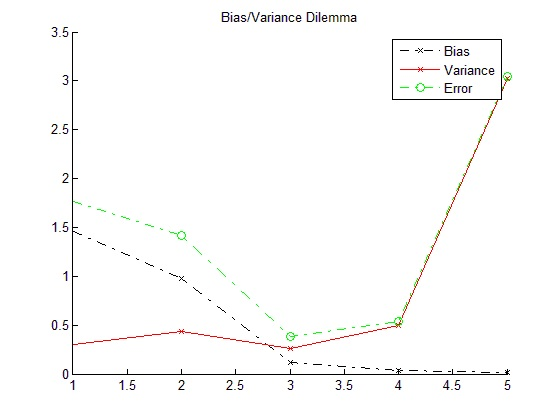I will be publishing the homeworks that I have done for Pattern Recognition course, I believe that they deal with important topics in Pattern Recognition and  someone may benefit from them.

For anyone that needs to get deep in the theory of Pattern Recognition and train themselves in the field I suggest our course book Introduction to Machine Learning by Ethem Alpaydin.

A quite general phenomenon arising in all kinds of pattern recognition methods is the dilemma between bias and variance. The bias and variance we are speaking of are two properties of our model that seem to have a conflicting relation. Whenever we fit a model to a data, we can notice two properties; bias, giving us a some kind of measure on our model’s predictions’ average closeness to training data and variance, deviation of the predictions by our model from the original data. If we fit a model with a low complexity (i.e. less assumptions),  our model’s predictions on data are usually very different from real values, do not follow the real trend in data and our model introduces high bias in its predictions and because of this high bias our model will not be able to follow the real trend in data and will produce predictions that are closer to our other predictions hence low variance. As we increase the complexity, bias of our model will decrease producing much better results on prediction however our predictions will be based more strongly on our input and we will be able to fit to individual points better but a prediction will deviate more from our other predictions, increasing variance.These contrasting trends for bias and variance is the cause for bias/variance dilemma. One may question why it is called a dilemma noting that our predictions get better with more complexity and model with the highest complexity is the choice for best performance. However, we should never lose sight of the fact that we are fitting a model to the available data and actually only performance on training data is getting better. As we increase the complexity of our models, there is a critical point where our model performs best on data that it is not trained on (test data). This point corresponds to the point where sum of bias and variance for our model reaches a minimum.

In this post, we will aim to observe the bias/variance dilemma in the context of polynomial regression. We will a generate a synthetic training data by producing samples by taking points on a known function and adding a random normal noise to them. Our input function: f(x)=2sin(1.5x) where x is uniform between 0 and 5 We will add random normal noise with mean 0 and standard deviation 1. We will generate 100 samples each containing i=1,…,20 points sampled as above. We will also generate a separate validation set containing 100 points without noise.

We will fit polynomials of degree 1 to 5 to training data and calculate their bias and variances.As it can be seen from the above plot, bias of the estimator decreases with degree of the polynomial fitted while variance of the estimator increases. For every different sample, when the polynomial degree increases, the polynomials fit to samples with less error. However, since the data is taken from a noisy training set, this also means that the fitted polynomials also learn the error which harms the flexibility and estimation power of the fitted models. Average fits get closer to actual function as the degree increases and the bias of models presents a declining trend. However, variance of models rises due to the fact that polynomials also learn the noise in the data set. Total error which is the sum of bias2 and variance reaches a minimum at degree 3 which is optimal degree for the given problem. For degrees under 3, model under-fits the data while for larger degrees it over-fits.